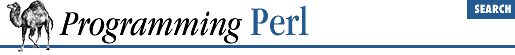home | O'Reilly's CD bookshelfs | FreeBSD | Linux | Cisco | Cisco Exam

#### 7.2.43 Math::BigInt - Arbitrary-Length Integer Math Package

```use Math::BigInt;

\$i = Math::BigInt->new(\$string);

# ```

BINT
```
is a big integer string; in all following cases \$i remains unchanged.
# All methods except bcmp() return a big integer string, or strings.
\$i->bneg;       # return negative of \$i
\$i->babs        # return absolute value of \$i
\$i->bcmp(```

BINT
```
)  # compare \$i to ```

BINT
```
; see below for return value
\$i->badd(```

BINT
```
)  # return sum of ```

BINT
```
and \$i
\$i->bsub(```

BINT
```
)  # return \$i minus ```

BINT
```

\$i->bmul(```

BINT
```
)  # return \$i multiplied by ```

BINT
```

\$i->bdiv(```

BINT
```
)  # return \$i divided by ```

BINT
```
; see below for return value
\$i->bmod(```

BINT
```
)  # return \$i modulus ```

BINT
```

\$i->bgcd(```

BINT
```
)  # return greatest common divisor of \$i and ```

BINT
```

\$i->bnorm       # return normalization of \$i```

This module allows you to use integers of arbitrary length. Integer strings (``` BINT ``` s) have the form ``` /^\s*[+-]?[\d\s]+\$/``` . Embedded whitespace is ignored. Output values are always in the canonical form: ``` /^[+-]\d+\$/``` . For example:

```'+0'                # canonical zero value
'   -123 123 123'   # canonical value:  '-123123123'
'1 23 456 7890'     # canonical value:  '+1234567890'```

The return value ``` NaN``` results when an input argument is not a number, or when a divide by zero is attempted. The ``` bcmp()``` method returns ``` -1``` , ``` 0``` , or ``` 1``` depending on whether ``` \$f``` is less than, equal to, or greater than the number string given as an argument. If the number string is undefined or null, the undefined value is returned. In a list context the ``` bdiv()``` method returns a two-element array containing the quotient of the division and the remainder; in a scalar context only the quotient is returned.

When you use this module, Perl's basic math operations are overloaded with routines from Math::BigInt. Therefore, you don't have to employ the methods shown above to multiply, divide, and so on. You can rely instead on the usual operators. Given this code:

```\$a = Math::BigInt->new("42 000 000 000 000");
\$b = Math::BigInt->new("-111111");```

the following five lines yield these string values for ``` \$c``` :

```\$c = 42000000000000 - -111111;
# 42000000111111; ordinary math--\$c is a double
\$c = \$a - \$b;             # "+42000000111111"; \$c is now a BigInt object
\$c = \$a - -111111;        # "+42000000111111"; \$c is now a BigInt object
\$c = \$a->bsub(\$b);        # "+42000000111111"; \$c is just a string
\$c = \$a->bsub(-111111);   # "+42000000111111"; \$c is just a string```7.2.42 Math::BigFloat - Arbitrary-Length, Floating-Point Math Package7.2.44 Math::Complex - Complex Numbers Package